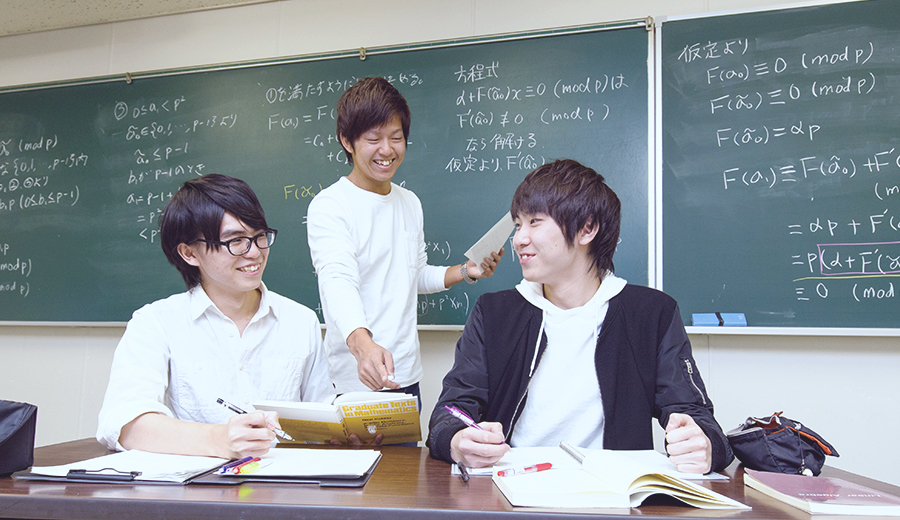# Mathematics Course, Department of Science

##### (a.k.a. Department of Mathematics)## Introduction

##### The Study of Mathematics—Universal and Timeless

From its origins in ancient Greece up to the present day, the field of mathematics has undergone a process of continuous evolution. All scientific technology is founded on areas of pure mathematics such as algebra, geometry, and mathematical analysis. Other elements of mathematics, including graph theory and cryptographic theory, play a critical role in sustaining today's highly network-oriented society. Established along with the Faculty of Science and Engineering 60 years ago, this course has a proven track record in providing effective advanced education. The teaching staff consists of leading mathematicians who deliver lectures based on the results of their latest research.

##### Instilling the Confidence to Take on New Challenges

This course aims to equip students with the knowledge and skills to solve problems by evaluating them in a logical and comprehensive way. For this reason, each seminar has a small class size, so that students can interact directly with instructors. Students also have ample opportunity to make presentations. This approach enables instructors to deliver lectures that match each student's level of understanding—something that would be difficult to achieve in conventional one-way lectures. In the third-year Study of Mathematics class for those contemplating a teaching career, third-year students gain practical mathematics teaching experience by assisting first-year students with their studies.

## Curriculum

##### Interactive Lectures Encompassing Pure and Applied Mathematics

Following a systematic introduction to pure mathematics—including algebra, geometry, and mathematical analysis—students investigate topics in applied mathematics, such as graph theory and cryptographic theory. In this course, we don't teach theory in a teacher-centered top-down fashion. Instead, we emphasize a one-to-one communication style that stimulates intellectual curiosity and equips students with a flexible and creative mindset.

#### curriculum

Specialized subjects 1st year 2nd year 3rd year 4th year
Required subjects Study of Mathematics (1) 
Study of Mathematics (2) 
Theory of Linear Algebra (1) 
Fundamental Analysis (1) 
Study of Mathematics (3) 
Study of Mathematics (4) 
Study of Mathematics (5) 
Study of Mathematics (6) 
Study of Mathematics (7) 
Study of Mathematics (8) 
Individual Study for Bachelor Thesis 
Elective required subjects       Modern Mathematics (1) 
Modern Mathematics (2) 
Modern Mathematics (3) 
Modern Mathematics (4) 
Modern Mathematics (5) 
Applied Mathematics (1) 
Applied Mathematics (2) 
Elective subjects Fundamental Geometry  Theory of Linear Algebra (2) 
Fundamental Analysis (2) 
Group Theory (1) 
Group Theory (2) 
Set Theory and Topology (1) 
Set Theory and Topology (2) 
Theory of Differential Equations (1) 
Theory of Differential Equations (2) 
Exercises in Computers (1) 
Complex Analysis (1) 
Complex Analysis (2) 
Algebra (1) 
Algebra (2) 
Geometry (1) 
Geometry (2) 
Real Analysis (1) 
Real Analysis (2) 
Mathematical Statistics (1) 
Mathematical Statistics (2) 
Exercises in Computers (2) 
Experimental Mathematics 

• This curriculum is for the year 2014 and is subject to change for 2015.
• Figures in [ ] indicate the number of credits.